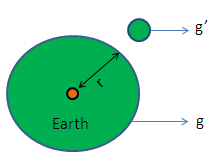# Acceleration Due to Gravity Calculation

The calculator calculates the occurrence of the acceleration due to gravity on exterior portion of the earth.

 Earth's surface (g): Earth's radius (re): Radius of outside the earth (r):

 Acceleration due to gravity (g'):

## Formula:

g' = ( re2 / r2) * g

Where,

g - Earth Surface,

r - Outside Radius of Earth,

g' - Acceleration due to gravity### Factors affecting acceleration due to gravity

The shape of the Earth.

Rotational motion of the Earth.

Altitude above the Earth's surface.

Depth below the Earth's surface.

The SI unit of acceleration is the metre per second squared (m s−2)